Next: 2.6 Area of a Up: 2. Some Area Calculations Previous: 2.4 Finite Geometric Series   Index

# 2.5 Area Under the Curve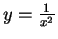The following argument is due to Pierre de Fermat (1601-1665) [19, pages 219-222]. Later we will use Fermat's method to find the area under the curve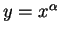for all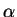in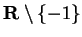.

Let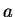be a real number with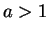, and let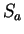be the set of points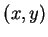in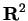such that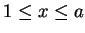and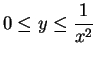. I want to find the area of.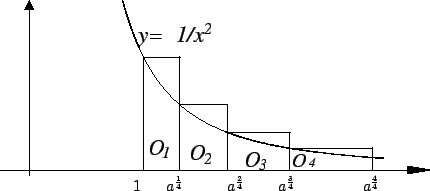Let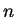be a positive integer. Note that since, we have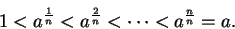Let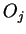be the box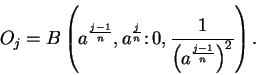Thus the upper left corner oflies on the curve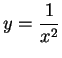.

To simplify the notation, I will write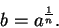Then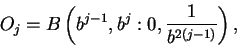and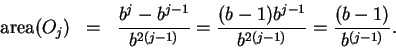Hence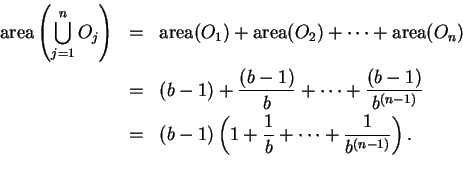Observe that we have here a finite geometric series, so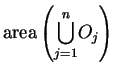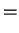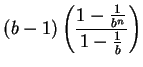(2.30)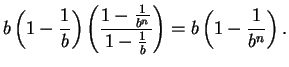(2.31)

Now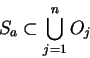(2.32)

so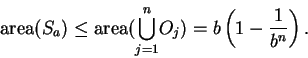(2.33)

Let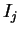be the box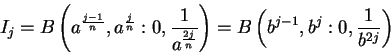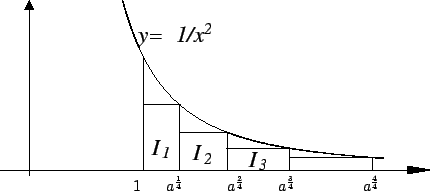so that the upper right corner oflies on the curveandlies underneath the curve. Then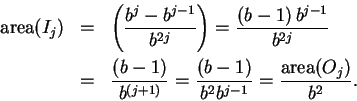Hence,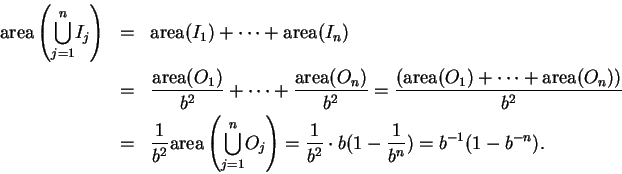Since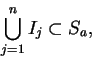we have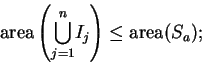i.e.,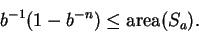By combining this result with (2.33), we get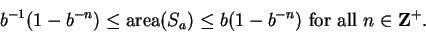Since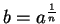, we can rewrite this as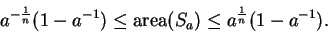(2.34)

2.35   Exercise. What do you think the area ofshould be? Explain your answer. If you have no ideas, take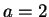in (2.34), take large values of, and by using a calculator, estimate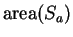to three or four decimal places of accuracy.

2.36   Exercise. A Letbe a real number with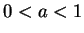, and let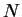be a positive integer. Then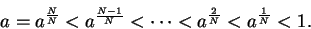Let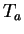be the set of pointssuch that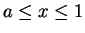and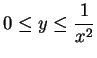. Draw a sketch of, and show that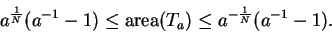The calculation of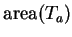is very similar to the calculation of.

What do you think the area ofshould be?

2.37   Exercise. Using the inequalities (2.6), and the results of Bernoulli's table in section 2.2, try to guess what the area of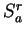is for an arbitrary positive integer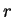. Explain the basis for your guess. ( The correct formula for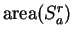for positive integerswas stated by Bonaventura Cavalieri in 1647[6, 122 ff]. Cavalieri also found a method for computing general positive integer power sums.)Next: 2.6 Area of a Up: 2. Some Area Calculations Previous: 2.4 Finite Geometric Series   Index
Ray Mayer 2007-09-07